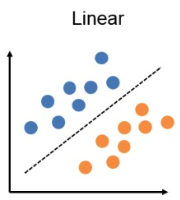# Artificial Neural Network

Back to glossary

## What is an Artificial Neural Network?

An artificial neuron network (ANN) is a computing system patterned after the operation of neurons in the human brain.

## How Do Artificial Neural Networks Work?

Artificial Neural Networks can be best viewed as weighted directed graphs, that are commonly organized in layers. These layers feature many nodes which imitate biological neurons of the human brain. that are interconnected and contain an activation function. The first layer receives the raw input signal from the external world-- analogous to optic nerves in human visual processing. Each successive layer gets the output from the layer preceding it, similar to the way neurons that are situated further from the optic nerve receive signals from those closest to them. The output at each node is called its activation or node value. The last tier produces the output of the system. ANNs are actually mathematical models that are capable of learning; by using ANNs we have been able to enhance existing data analysis technologies. They are one of the reasons we have seen an important progress in artificial intelligence (AI), machine learning (ML), and deep learning.

## Perceptron Artificial Neural Network

Perceptron is the simplest type of artificial neural network. This type of network is typically used for making binary predictions. A Perceptron can only work if the data can be linearly separable.## Multi-layer Artificial Neural Network

A fully connected multi-layer neural network is also known as a Multilayer Perceptron (MLP). This type of artificial neural network is made of more than one layer of artificial neurons or nodes, ( for example the Convolutional Neural Network, Recurrent Neural Network etc …) A multi-layer ANN is used to solve more complex classification and regression tasks. The most common model is the 3-layer fully-connected backpropagation model. The first layer consists of input neurons, that send data on to the second layer, which in turn sends the output neurons to the third layer.Furthermore, there are two Artificial Neural Network topologies: FeedForward and Feedback.

## FeedForward Artificial Neural Network

In this ANN, the information flow is unidirectional. The information travels only in one direction; forward; without making any feedback loops.  It first goes through the input nodes, then through the hidden nodes (in case there are any), and in the end, it goes through the output nodes.

## FeedBack Artificial Neural Network

In this case, there are inherent feedback connections between the neurons of the networks. Here, feedback loops are allowed.